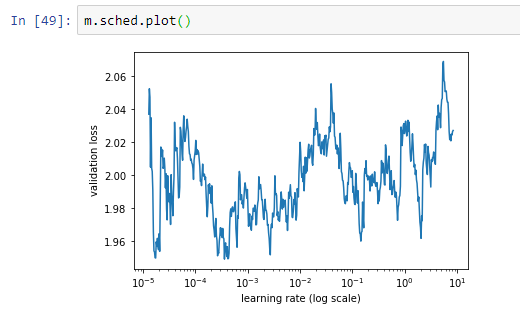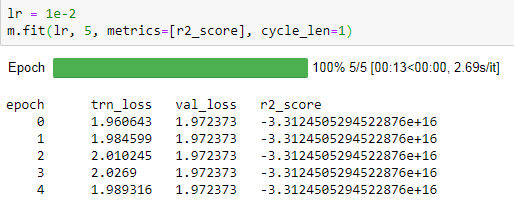# Using Rossman approach for housing dataset

I recently finished part 1 of the deep learning course and tried to predict housing prices at this competition – https://www.kaggle.com/c/house-prices-advanced-regression-techniques

The data set contains 1460 rows with 80 parameters, the test set is about the same size. I used the Rossman lesson code, making relevant modifications. Here’s what I came up with: https://gist.github.com/adsalpha/409aafd578a1f675d0053c29f332a9ea. This placed 1976 on the public leaderboard.

1. Could you recommend what to adjust in order to make it more accurate?

2. I observed some strange behavior when attempting to use a different learning rate than `1e-3` (the LR finder showed that something like `5 * 1e-3` would suit better). The loss functions for the training and validation set start showing excessively high numbers which remain pretty much constant no matter how many times I fit. The thing from below persists on all calls of `.fit`. When I use `1e-3` everything is OK. What is the cause of this behavior, is it expected?

``````epoch      trn_loss   val_loss
0      18.026798  18.181596
1      18.008082  18.181596
2      18.131493  18.181596``````
1 Like

I’ve noticed the same thing when training on my own data. Not sure why, but feels like the model at that specific architecture and lr gets stuck in some sort of horrible area. Or maybe it’s how it’s initialized, not sure.

Also, this method I’ve found depends a lot on the ‘width’ of the categorical variables. I’ve found that when my variables have very little number of categories I can get better performance for allowing larger embeddings. Probably not the best idea but it works.

Also, try using this before fit and let me know if it does better.

1. First create your training phases, I define 4 of them here starting with RMSprop and switching to adamW, use 1-cycle with differential learning rates. Remember to change the settings to fit the number of layers you’ve assigned. Feel free to play around with the settings. Also, try to see when your model overfits and run until there, performance always seems to deteriorate when training loss gets much lower than validation.

def phases_1cycle_discriminative(cycle_len,lr,div,wds, pct,max_mom,min_mom):
…lrs = np.array([lr/100, lr/10, lr])
…return [TrainingPhase(epochs=(cycle_len * (1-pct) / 4), opt_fn=optim.RMSprop, lr=(lrs/div,lrs), lr_decay=DecayType.LINEAR,
momentum=(max_mom,min_mom), momentum_decay=DecayType.LINEAR, wd_loss=False),
TrainingPhase(epochs=(cycle_len * (1-pct) / 2), opt_fn=optim.Adam, lr=lrs, lr_decay=DecayType.NO,
momentum=min_mom, momentum_decay=DecayType.NO, wds=wds, wd_loss=False),
TrainingPhase(epochs=(cycle_len * (1-pct) / 4), opt_fn=optim.Adam, lr=(lrs,lrs/div),
wds=wds, wd_loss=False, lr_decay=DecayType.LINEAR, momentum=(min_mom,max_mom),
momentum_decay=DecayType.LINEAR),
TrainingPhase(epochs=(cycle_len * pct), opt_fn=optim.Adam, lr=(lrs/div, lrs/div), wds=wds, wd_loss=False,
lr_decay=DecayType.COSINE, momentum=max_mom, momentum_decay=DecayType.NO)]

1. After that you can just call the functon and create your training phases (change to your learning rate here):

phases = phases_1cycle_discriminative(cycle_len=8, lr=5e-3, div=10, wds=1.26e-7, pct=0.1, max_mom=0.99, min_mom=0.85)

1. After that all you have to do is fit your model, with one more extra fastai awesomeness: stochastic weight averaging:

m.fit_opt_sched(phases, use_swa=True, swa_start=3)

1. You can visualize your learning rate and momentum schedule with the following command:

m.sched.plot_lr(show_text=False, show_moms=True)

That’s all! Let me know if that helps! In any case, it’s fun using fastai’s arsenal.

Regards,
Theodore.

P.S.: I don’t take any credit for the above, it’s all based on @sgugger’s TrainingPhase API.

2 Likes

I’m running in to a very similar problem with a dataset from work. I tried the above code adjusting the training phase and that didn’t have any impact.

The dataset is ~50,000 rows by 20 columns, half of which are categorical.

Below is the plot from the learning rate finder:And then the model fit widget shows this:I’m not really sure where to go from here and hoping you all might have some ideas of things to try next. Thanks.

It would really help if you give more info, and maybe move to fastai V1.

I have been trying to use the Tabular tutorial for the Rossman dataset and have been unable to successfully create a TabularDataBunch. If I used a valid_idx less than the full length of the dataset and include categories with uncommon variables I get the error

`Exception: Your validation data contains a label that isn't present in the training set, please fix your data.` and if I limit my data subset I get `AttributeError: 'TabularList' object has no attribute 'codes'`

Two questions: How is the attribute ‘codes’ set and what does this error mean, and can I pass a df that I create via sklearn to avoid the validation errors with uncommon variables?

data = TabularDataBunch.from_df(path, df, dep_var, valid_idx=valid_idx, procs=procs, cat_nam. es=cat_names)